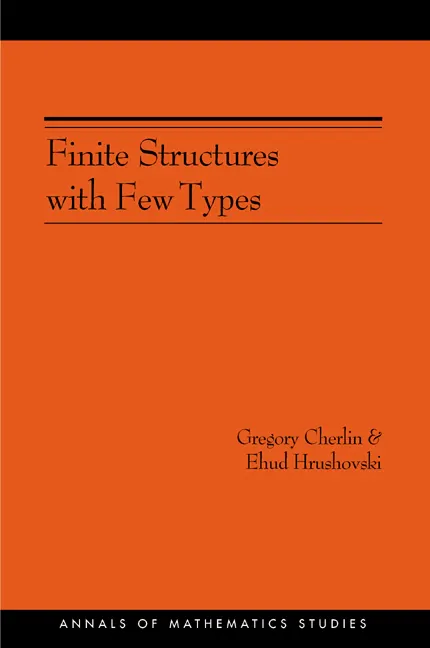Gregory Cherlin

# Finite Structures with Few Types. (AM-152), Volume 152## Paperback

Price:
\$81.00/£68.00
ISBN:
Published:
Jan 12, 2003
2003
Pages:
200
Size:
6 x 9.25 in.

This book applies model theoretic methods to the study of certain finite permutation groups, the automorphism groups of structures for a fixed finite language with a bounded number of orbits on 4-tuples. Primitive permutation groups of this type have been classified by Kantor, Liebeck, and Macpherson, using the classification of the finite simple groups.

Building on this work, Gregory Cherlin and Ehud Hrushovski here treat the general case by developing analogs of the model theoretic methods of geometric stability theory. The work lies at the juncture of permutation group theory, model theory, classical geometries, and combinatorics.

The principal results are finite theorems, an associated analysis of computational issues, and an “intrinsic” characterization of the permutation groups (or finite structures) under consideration. The main finiteness theorem shows that the structures under consideration fall naturally into finitely many families, with each family parametrized by finitely many numerical invariants (dimensions of associated coordinating geometries).

The authors provide a case study in the extension of methods of stable model theory to a nonstable context, related to work on Shelah’s “simple theories.” They also generalize Lachlan’s results on stable homogeneous structures for finite relational languages, solving problems of effectivity left open by that case. Their methods involve the analysis of groups interpretable in these structures, an analog of Zilber’s envelopes, and the combinatorics of the underlying geometries. Taking geometric stability theory into new territory, this book is for mathematicians interested in model theory and group theory.